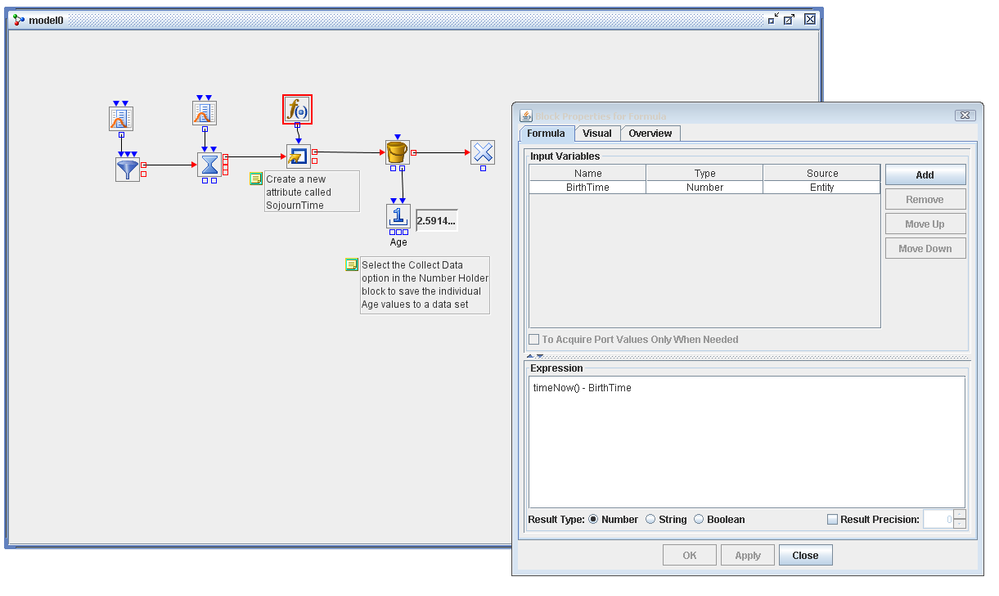## Calculation of sojourn time in SAS Simulation Studio

Hello,

I am using SAS Simulation Studio 13.2 for developing service business model.

In the simulation process, I would like to track sojourn time of every entities and assign different attibute.

I am new to SAS sim studio, so I do not know how to calculate sojourn time (I guess birth time of entity is automatically assigned, but couldn't find the way to see sojourn time (= current time - birth time)).

Please let me know how to calculate sojourn time.

1 ACCEPTED SOLUTION

Accepted Solutions

## Re: Calculation of sojourn time in SAS Simulation Studio

There are a couple of different options that you can calculate the time in system in Simulation Studio.  First, the Bucket block will automatically calculate a value called Age for each entity that passes through it.  For each entity that passes through the Bucket, a row is added to a data model and the columns of that data model are the entity attribute values (including Age).  You can write the contents of the Bucket to a SAS data set.  The Bucket also has an OutAge port that allows you to pass the Age value to another place in the model.  In the attached simple model, the OutAge port of the Bucket is connected to a Number Holder block with the Collect Data option selected.  The Number Holder block can then be used to save only the Age attribute values.

One other option is to manually compute the sojourn time using the Formula block and then assign the value to an entity attribute using a Modifier block.  There is a default attribute called BirthTime that each entity is assigned when it is created.   In the attached screenshot, the Formula used to calculate the SojournTime is shown.2 REPLIES 2

## Re: Calculation of sojourn time in SAS Simulation Studio

There are a couple of different options that you can calculate the time in system in Simulation Studio.  First, the Bucket block will automatically calculate a value called Age for each entity that passes through it.  For each entity that passes through the Bucket, a row is added to a data model and the columns of that data model are the entity attribute values (including Age).  You can write the contents of the Bucket to a SAS data set.  The Bucket also has an OutAge port that allows you to pass the Age value to another place in the model.  In the attached simple model, the OutAge port of the Bucket is connected to a Number Holder block with the Collect Data option selected.  The Number Holder block can then be used to save only the Age attribute values.

One other option is to manually compute the sojourn time using the Formula block and then assign the value to an entity attribute using a Modifier block.  There is a default attribute called BirthTime that each entity is assigned when it is created.   In the attached screenshot, the Formula used to calculate the SojournTime is shown.## Re: Calculation of sojourn time in SAS Simulation Studio

Thank you Emily.

Discussion stats
• 2 replies
• 920 views
• 0 likes
• 2 in conversation